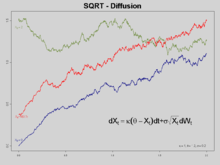# Cox–Ingersoll–Ross model

﻿
Cox–Ingersoll–Ross modelThree trajectories of CIR Processes

In mathematical finance, the Cox–Ingersoll–Ross model (or CIR model) describes the evolution of interest rates. It is a type of "one factor model" (short rate model) as it describes interest rate movements as driven by only one source of market risk. The model can be used in the valuation of interest rate derivatives. It was introduced in 1985 by John C. Cox, Jonathan E. Ingersoll and Stephen A. Ross as an extension of the Vasicek model.

## The model

The CIR model specifies that the instantaneous interest rate follows the stochastic differential equation, also named the CIR process:$dr_t = a(b-r_t)\, dt + \sigma\sqrt{r_t}\, dW_t$

where Wt is a Wiener process modelling the random market risk factor.

The drift factor, a(b − rt), is exactly the same as in the Vasicek model. It ensures mean reversion of the interest rate towards the long run value b, with speed of adjustment governed by the strictly positive parameter a.

The standard deviation factor,$\sigma \sqrt{r_t}$, avoids the possibility of negative interest rates for all positive values of a and b. An interest rate of zero is also precluded if the condition$2 a b \geq \sigma^2 \,$

is met. More generally, when the rate is at a low level (close to zero), the standard deviation also becomes close to zero, which dampens the effect of the random shock on the rate. Consequently, when the rate gets close to zero, its evolution becomes dominated by the drift factor, which pushes the rate upwards (towards equilibrium).

## Bond pricing

Under the no-arbitrage assumption, a bond may be priced using this interest rate process. The bond price is exponential affine in the interest rate:$B(t,T) = \exp(A(t,T) + r(t) C(t,T))\!$

## Extensions

Time varying functions replacing coefficients can be introduced in the model in order to make it consistent with a pre-assigned term structure of interest rates and possibly volatilities. The most general approach is in Maghsoodi (1996). A more tractable approach is in Brigo and Mercurio (2001b) where an external time-dependent shift is added to the model for consistency with an input term structure of rates. A significant extension of the CIR model to the case of stochastic mean and stochastic volatility is given by Lin Chen(1996) and is known as Chen model. A CIR process is a special case of a basic affine jump diffusion, which still permits a closed-form expression for bond prices.

Wikimedia Foundation. 2010.

### Look at other dictionaries:

• Cox-Ingersoll-Ross model — The Cox Ingersoll Ross model in finance is a mathematical model describing the evolution of interest rates. It is a type of one factor model (Short rate model) as describes interest rate movements as driven by only one source of market risk. The… …   Wikipedia

• Short rate model — In the context of interest rate derivatives, a short rate model is a mathematical model that describes the future evolution of interest rates by describing the future evolution of the short rate.The short rateThe short rate, usually written r t… …   Wikipedia

• Vasicek model — In finance, the Vasicek model is a mathematical model describing the evolution of interest rates. It is a type of one factor model (short rate model) as it describes interest rate movements as driven by only one source of market risk. The model… …   Wikipedia

• Stephen Ross (economist) — Stephen Alan Steve Ross is the inaugural Franco Modigliani Professor of Financial Economics at the MIT Sloan School of Management. He is known for initiating several important theories and models in financial economics. He is a widely published… …   Wikipedia

• John Carrington Cox — is the Nomura Professor of Finance at the MIT Sloan School of Management. He is one of the world s leading experts on options theory and one of the inventors of the Cox Ross Rubinstein model for option pricing, as well as of the Cox Ingersoll… …   Wikipedia

• Jonathan E. Ingersoll — Jonathan Edwards Jon Ingersoll, Jr. is an American economist. He is currently the Adrian C. Israel Professor at Yale School of Management, having previously taught at the University of Chicago.Jonathan Ingersoll s research area is finance,… …   Wikipedia

• Stephen Ross — Stephen Alan Ross (* 1944) ist ein US amerikanischer Wirtschaftswissenschaftler und Finanzmathematiker. Sein Name ist eng verbunden mit der Entwicklung der Arbitrage und der Optionspreistheorie. Werdegang, Forschung und Lehre Ross studierte… …   Deutsch Wikipedia

• CIR process — The CIR process (named after its creators John C. Cox, Jonathan E. Ingersoll, and Stephen A. Ross) is a Markov process with continuous paths defined by the following stochastic differential equation (SDE): where Wt is a standard Wiener process… …   Wikipedia

• List of finance topics — Topics in finance include:Fundamental financial concepts* Finance an overview ** Arbitrage ** Capital (economics) ** Capital asset pricing model ** Cash flow ** Cash flow matching ** Debt *** Default *** Consumer debt *** Debt consolidation ***… …   Wikipedia

• Outline of finance — The following outline is provided as an overview of and topical guide to finance: Finance – addresses the ways in which individuals, businesses and organizations raise, allocate and use monetary resources over time, taking into account the risks… …   Wikipedia